Next: Late-breaking News ... Up: Complexity Previous: Fixed Point Free Automorphisms

## Xia's Reduction Algorithm

Xia's algorithm (cf. ), a variant of which we are about to describe, terminates in polynomial time. If it terminates with a graph with no edges, the ordered set is known to have the fixed point property (cf. Theorem 2.22). The main idea is that certain combinations of elements incannot be contained in an order-preserving map (cf. Lemma 2.19). Formulating our results in the language of graph theory it seems that the ``natural habitat" of Xia's algorithm is the decision if a given r-partite graph G=(V,E) with partition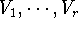and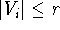contains an r-clique.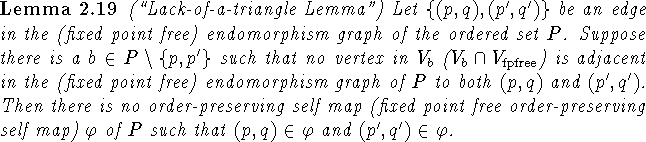Proof: This is obvious, as otherwise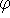would contain a complete induced subgraph with vertices (p,q), (p',q'), (b,c) (a ``triangle") for some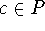(resp. some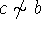). \

The Lack-of-a-triangle Lemma now suggests the following removal step which is decribed as step II in  on p. 260:

 REMOVE Let G=(V,E) be a subgraph of the   (fixed point free) endomorphism graph. Find an edge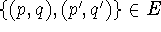such that there is a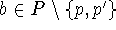such that no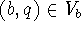(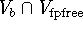) is adjacent in G to both (p,q) and (p',q'). Remove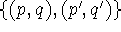from E to obtain a new graph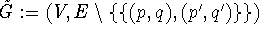. If this cannot be done, stop.

Xia's algorithm now iterates this removal step until the iteration is no longer possible.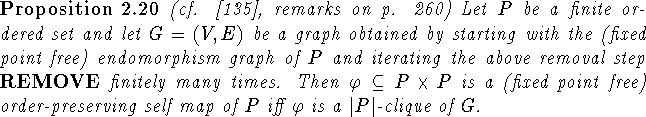Proof: As G is a subgraph of the endomorphism graph of P, the direction ``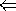" is clear. To prove ``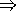", we perform an induction on n, the number of iterations of the removal steps. For n=0 the result is true by Corollary 2.9. Now assume that G=(V,E) has been obtained from the (fixed point free) endomorphism graph through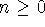iterations of the removal step and that if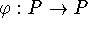is an order-preserving self map of P thenis a |P|-clique of G. Let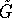be the graph obtained in the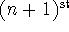iteration of the removal step and letbe an order-preserving self map. Then the removed edge cannot be an edge that connects two elements of. Thus the conclusion holds in. \

Proposition 2.20 shows that iteration of the removal step ``does not destroy any (fixed point free) self maps". Thus if this iteration eventually produces a graph with an empty edge set, then P must have the fixed point property. Obviously this suggested algorithm is polynomial. Moreover Proposition 2.21 shows that the graph obtained by iterating the removal step until no further edges can be removed is independent of the order in which edges are removed resp. independent of the search pattern used in REMOVE.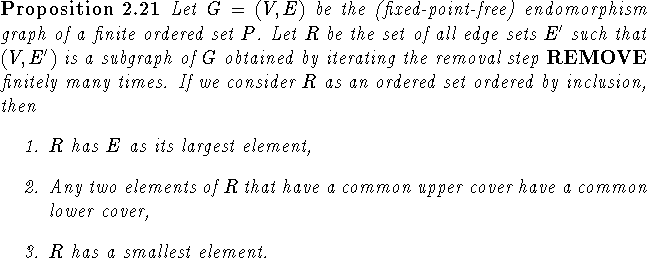Proof: Part 1 is obvious and part 3 easily follows from the fact that a finite ordered set that satisfies 2 and has a largest element also has a smallest element (cf. ; Farley's argument in  obviously is the template for this proof). Thus we are left to prove part 2. Note that F is an upper cover of F' in R iff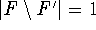and F' is obtained from F by applying the removal step once. Now suppose that A,B are in R and U is an upper cover of A and B. Let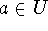be the edge such that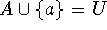and let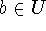be such that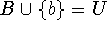. Then the removal step can be applied to U to remove a or b (whichever is found first) and hence the removal step can be applied to A to remove b (find b and remove it) and it can be applied to B to remove a (find a and remove it). Thus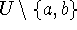is a lower cover of A and B in R. \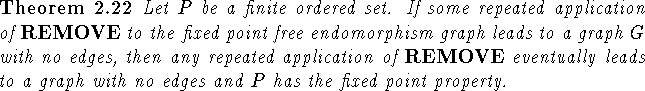Proof: By Proposition 2.21 REMOVE will eventually lead to a graph with no edges independent of the specific iteration being used. By Proposition 2.20 P has no fixed point free order-preserving map, since G has no |P|-clique. \

At the end of  it is proved that Xia's algorithm terminates with a graph with no edges when P is dismantlable (cf. Example 3.9, part 1). This is not surprising, as dismantlability can be checked in polynomial time and implies the fixed point property. Little is known about for what classes of ordered sets termination of Xia's algorithm with a graph that has edges guarantees the existence of a fixed point free self map (cf. open question 1). It is easy to show that Xia's algorithm terminates with a graph with no edges for connectedly collapsible ordered sets (cf. Definition 4.26) of height 2 and a modification of the proof in  shows the same is true for ordered sets with the cutset property.Next: Late-breaking News ... Up: Complexity Previous: Fixed Point Free Automorphisms

Bernd.S.W.Schroder